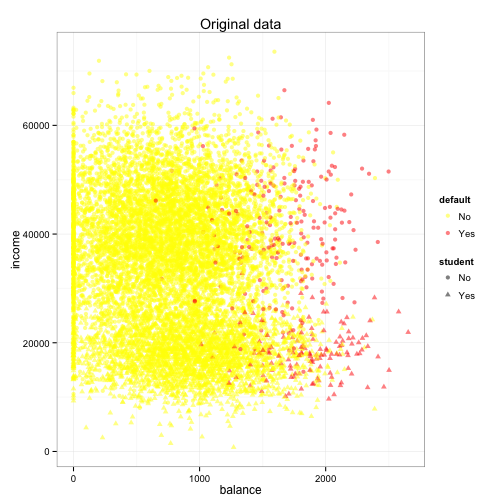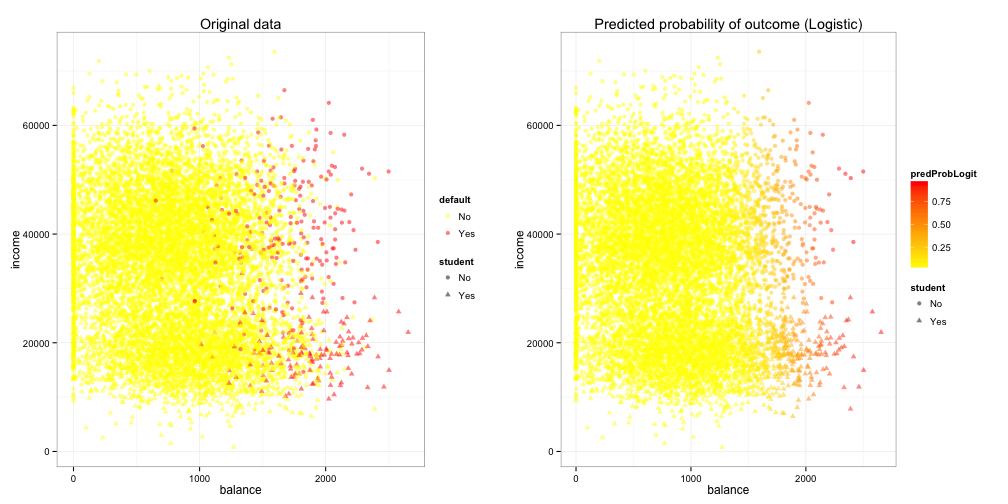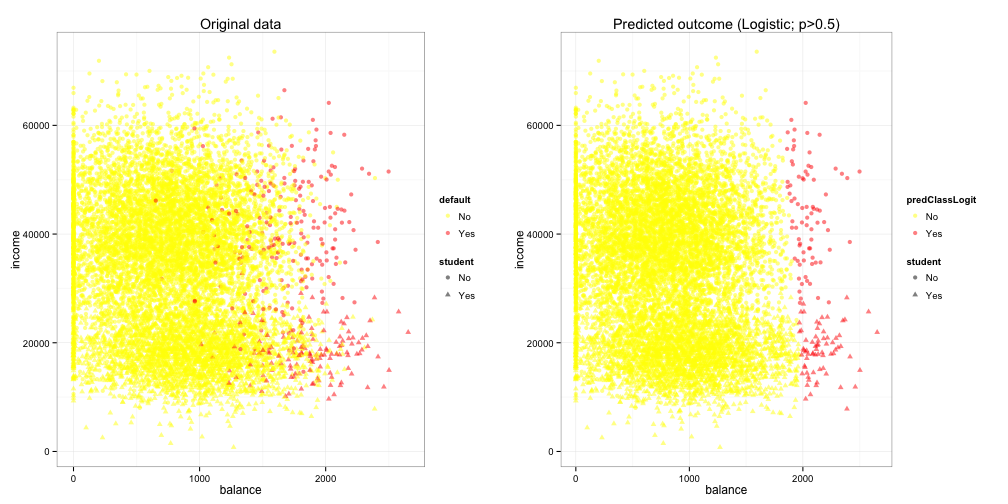# Linear discriminant analysis.

## References

• James et al. An Introduction to Statistical Learning with Applications in R

``````## for lda() and qda()
library(MASS)
## Data for An Introduction to Statistical Learning with Applications in R
library(ISLR)
## Plotting
library(ggplot2)
library(gridExtra)
## for knn()
library(class)
``````

``````data(package = "ISLR")
``````
``````Data sets in package ‘ISLR’:

Auto                               Auto Data Set
Caravan                            The Insurance Company (TIC) Benchmark
Carseats                           Sales of Child Car Seats
College                            U.S. News and World Report's College Data'
Default                            Credit Card Default Data
Hitters                            Baseball Data
Khan                               Khan Gene Data
NCI60                              NCI 60 Data
OJ                                 Orange Juice Data
Portfolio                          Portfolio Data
Smarket                            S&P Stock Market Data
Wage                               Mid-Atlantic Wage Data
Weekly                             Weekly S&P Stock Market Data
``````
``````## Load Default (Credit Card Default Data)
data(Default)
``````
``````##   default student balance income
## 1      No      No   729.5  44362
## 2      No     Yes   817.2  12106
## 3      No      No  1073.5  31767
## 4      No      No   529.3  35704
## 5      No      No   785.7  38463
## 6      No     Yes   919.6   7492
``````
``````Description:
A simulated data set containing information on ten thousand
customers. The aim here is to predict which customers will default
on their credit card debt.
Format:
A data frame with 10000 observations on the following 4 variables.
‘default’ A factor with levels ‘No’ and ‘Yes’ indicating whether
the customer defaulted on their debt
‘student’ A factor with levels ‘No’ and ‘Yes’ indicating whether
the customer is a student
‘balance’ The average balance that the customer has remaining on
their credit card after making their monthly payment
‘income’ Income of customer
``````

## Discriptive

Individuals with higher credit card balance may be more likely to default.

``````## Summary
summary(Default)
``````
``````##  default    student       balance         income
##  No :9667   No :7056   Min.   :   0   Min.   :  772
##  Yes: 333   Yes:2944   1st Qu.: 482   1st Qu.:21340
##                        Median : 824   Median :34553
##                        Mean   : 835   Mean   :33517
##                        3rd Qu.:1166   3rd Qu.:43808
##                        Max.   :2654   Max.   :73554
``````
``````## Plot
plotData <- ggplot(data = Default,
mapping = aes(x = balance, y = income, color = default, shape = student)) +
layer(geom = "point", stat = "identity", alpha = 0.5) +
scale_color_manual(values = c("No" = "yellow", "Yes" = "red")) +
theme_bw() +
theme(legend.key = element_blank()) +
labs(title = "Original data")
plotData
``````## Logistic Regression

Both the balance and the income variables are standardized for interpretability. Both factors are significant, but the coefficient is less impressive for the income variable. The color of the plot is arbitrary, the threshold (predicted probability > 0.5 is used) for deciding the predicted classification is up to the investigator.

``````## Fit logistic regression
resLogit <- glm(formula = default ~ scale(balance) + scale(income),
data    = Default)
## Show the result
summary(resLogit)
``````
``````##
## Call:
## glm(formula = default ~ scale(balance) + scale(income), family = binomial(link = "logit"),
##     data = Default)
##
## Deviance Residuals:
##    Min      1Q  Median      3Q     Max
## -2.473  -0.144  -0.057  -0.021   3.724
##
## Coefficients:
##                Estimate Std. Error z value Pr(>|z|)
## (Intercept)     -6.1256     0.1876  -32.66  < 2e-16 ***
## scale(balance)   2.7316     0.1100   24.84  < 2e-16 ***
## scale(income)    0.2775     0.0665    4.17  0.00003 ***
## ---
## Signif. codes:  0 '***' 0.001 '**' 0.01 '*' 0.05 '.' 0.1 ' ' 1
##
## (Dispersion parameter for binomial family taken to be 1)
##
##     Null deviance: 2920.6  on 9999  degrees of freedom
## Residual deviance: 1579.0  on 9997  degrees of freedom
## AIC: 1585
##
## Number of Fisher Scoring iterations: 8
``````
``````
## Put the predicted probability
Default\$predProbLogit <- predict(resLogit, type = "response")
Default\$predClassLogit <- factor(predict(resLogit, type = "response") > 0.5,
levels = c(FALSE,TRUE), labels = c("No","Yes"))

## Plot (probability)
plotLogit <- ggplot(data = Default,
mapping = aes(x = balance, y = income, color = predProbLogit, shape = student)) +
layer(geom = "point", alpha = 0.5) +
scale_color_gradient(low = "yellow", high = "red") +
theme_bw() +
theme(legend.key = element_blank()) +
labs(title = "Predicted probability of outcome (Logistic)")
grid.arrange(plotData, plotLogit, ncol = 2)
````````````
## Plot (classification)
plotLdaClass <- ggplot(data = Default,
mapping = aes(x = balance, y = income, color = predClassLogit, shape = student)) +
layer(geom = "point", alpha = 0.5) +
scale_color_manual(values = c("No" = "yellow", "Yes" = "red")) +
theme_bw() +
theme(legend.key = element_blank()) +
labs(title = "Predicted outcome (Logistic; p>0.5)")
grid.arrange(plotData, plotLdaClass, ncol = 2)
``````## Linear Discriminant Analysis

LDA is similar to logistic regression in this kind of a simple case. However, there are certain advantages.

• When the claases are well-separated, the logistic regression model is unstable. The LDA model does not have this problem, and actually performs well.
• When the sample size is small, as long as the predictors are normally distributed within each outcome category, the LDA model is more stable than the logistic regression model.
• When the outcome is multinominal, the LDA model is more popular and more interpretable.
``````## Fit LDA
resLda <- lda(formula = default ~ scale(balance) + scale(income),
data    = Default)
resLda
``````
``````## Call:
## lda(default ~ scale(balance) + scale(income), data = Default)
##
## Prior probabilities of groups:
##     No    Yes
## 0.9667 0.0333
##
## Group means:
##     scale(balance) scale(income)
## No        -0.06498      0.003688
## Yes        1.88633     -0.107061
##
## Coefficients of linear discriminants:
##                   LD1
## scale(balance) 1.0791
## scale(income)  0.1039
``````
``````
## Predict
predLda <- predict(resLda)
str(predLda)
``````
``````## List of 3
##  \$ class    : Factor w/ 2 levels "No","Yes": 1 1 1 1 1 1 1 1 1 1 ...
##  \$ posterior: num [1:10000, 1:2] 0.997 0.997 0.987 0.999 0.996 ...
##   ..- attr(*, "dimnames")=List of 2
##   .. ..\$ : chr [1:10000] "1" "2" "3" "4" ...
##   .. ..\$ : chr [1:2] "No" "Yes"
##  \$ x        : num [1:10000, 1] -0.1516 -0.2075 0.5177 -0.6659 -0.0724 ...
##   ..- attr(*, "dimnames")=List of 2
##   .. ..\$ : chr [1:10000] "1" "2" "3" "4" ...
##   .. ..\$ : chr "LD1"
``````
``````
## Put into the dataset
Default\$predProbLda <- predLda\$posterior[,"Yes"]
Default\$predClassLda <- predLda\$class

## Plot (probability)
plotLdaProb <- ggplot(data = Default,
mapping = aes(x = balance, y = income, color = predProbLda, shape = student)) +
layer(geom = "point", alpha = 0.5) +
scale_color_gradient(low = "yellow", high = "red") +
theme_bw() +
theme(legend.key = element_blank()) +
labs(title = "Predicted probability of outcome (LDA)")
grid.arrange(plotData, plotLdaProb, ncol = 2)
``````